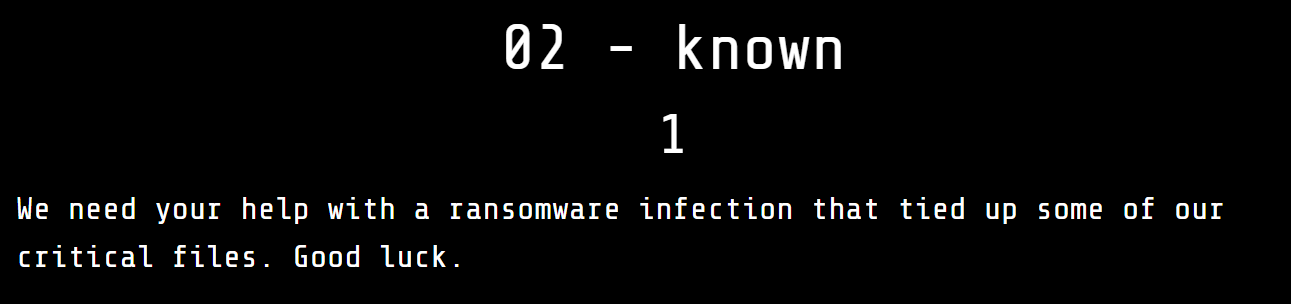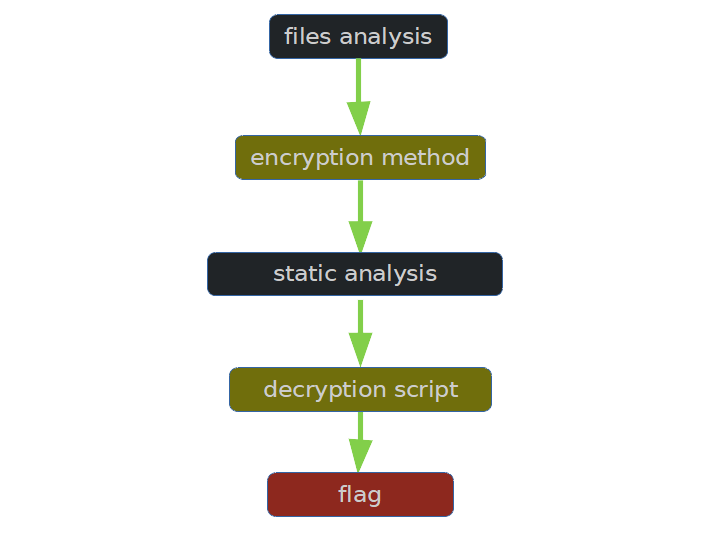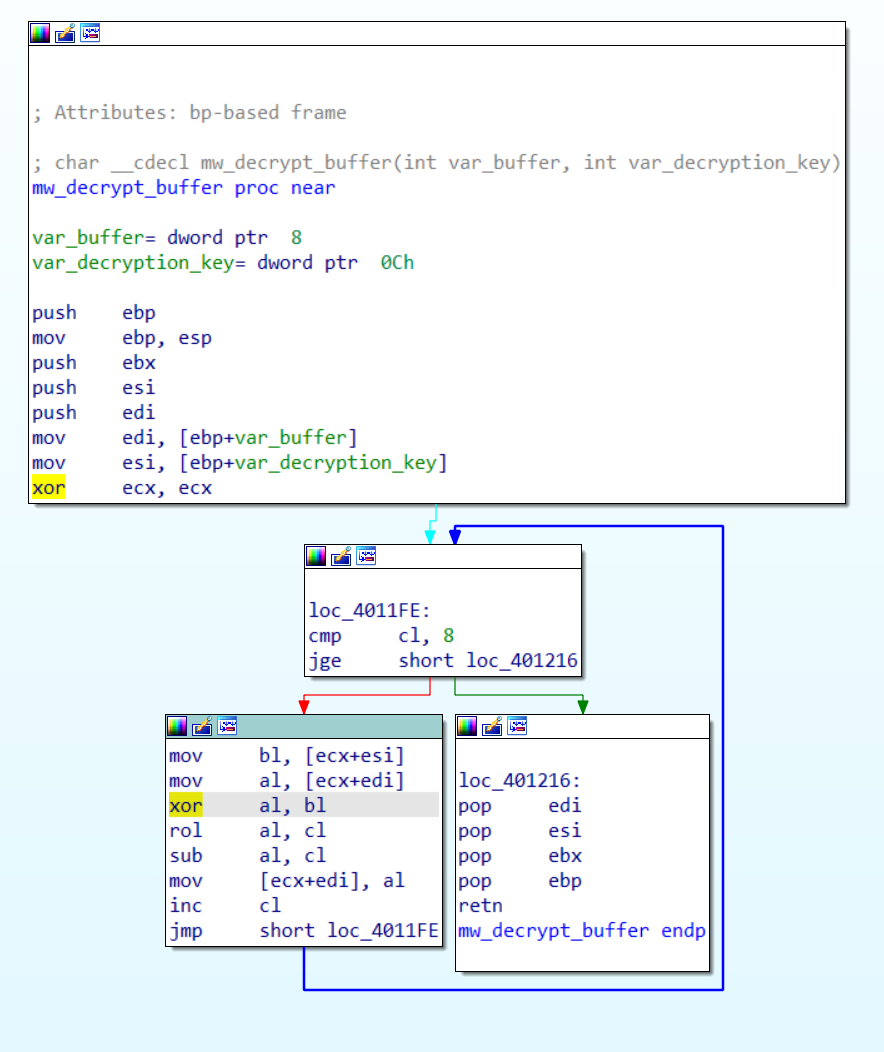# Writeup: FlareOn 2021: 002 - known## 1. TLDR## 2. Input data

The challenge file is here. Password: flare.

The available files are:

``````  UnlockYourFiles.exe
Files/capa.png.encrypted
Files/cicero.txt.encrypted
Files/commandovm.gif.encrypted
Files/critical_data.txt.encrypted
Files/flarevm.jpg.encrypted
Files/latin_alphabet.txt.encrypted
``````

## 3. File analysis

In the file we find a base64 encoded string that is a hint:

``````\$ echo KD4wXzApPiBJdCdzIGRhbmdlcm91cyB0byBh
ZGQrcm9yIGFsb25lISBUYWtlIHRoaXMgPCgwXzA8KQo= | base64 -d
(>0_0)> It's dangerous to add+ror alone! Take this <(0_0<)
``````

## 4. Code analysis

We find the function responsible for decrypting a packet of 8 bytes:The decryption algorithm was implemented as follows:

``````p[i] = rol(key[i] ^ c[i], i) - i
``````

The transformation yields an encryption algorithm:

``````c[i] =  ror(p[i] + i, i) ^ key[i]
``````

It is therefore necessary to recover the 8-byte key

## 5. Reading the flag

We know from the file names that one of the encrypted files contains the Latin alphabet. We can therefore find the key by looking for one that corresponded to a known ciphertext.

I therefore implemented an encryption function:

``````
def enrypt(plaintext, key):
max_bits = 8
ror = lambda val, r_bits, max_bits: \
((val & (2**max_bits-1)) >> r_bits%max_bits) | \
(val << (max_bits-(r_bits%max_bits)) & (2**max_bits-1))
i = 0
ciphertext = []
for (p,k) in zip(plaintext, key):
c = ror(ord(p) + (i%8), (i%8), max_bits) ^ k
ciphertext.append(c)
i = i+1
return ciphertext

``````

I then wrote code that finds the next bytes of the key:

``````def read_first_qword_buffer(filename):
buffer = None
with open(filename, 'rb') as f:
return buffer

key = []
ciphertext = None

for i in range(0,8):
for key_char_candidate in range(0,256):
key_candidate = key + [key_char_candidate]
ciphertext = enrypt('ABCDEFGH', key_candidate)
if all(a==b for (a,b) in zip(ciphertext, encrypted_file_header)):
key = key_candidate
break

print(''.join([chr(k) for k in key ]))
``````

As a result of the script, the keys were recovered:

``````No1Trust
``````

After running the UnlockYourFiles.exe program and entering the key, the encrypted files were recovered:

``````  Files/capa.png
Files/cicero.txt
Files/commandovm.gif
Files/critical_data.txt
Files/flarevm.jpg
Files/latin_alphabet.txt
``````

In the file `critical_data.txt` there was a flag:

``````(>0_0)> You_Have_Awakened_Me_Too_Soon_EXE@flare-on.com <(0_0<)
``````